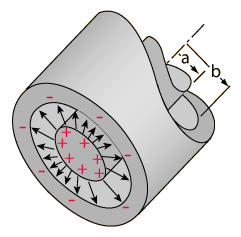## Cylindrical Capacitor Calculator

 I want to calculate --Select-- Permittivity Length of Conductors Outer Conductor Diameter Inner Conductor Diameter Capacitance Permittivity (ε): F/m Length of Conductors (L): m Outer Conductor Diameter (b): m Inner Conductor Diameter (a): m Capacitance (C): F

### Cylindrical Capacitor Formula

To Inner Conductor Diameter : a = b/e (2π L/C)

To Outer Conductor Diameter : b = ae (2πL/C)

Length of Conductors : L = Cln(b/a)/2π ε

To Permittivity : ε = Cln (b/a)/2πL

To Capacitance : C = 2π εL/In( b/a)

Where, ε = Permittivity, C = Capacitance, b = Outer Conductor Diameter, L = Length of Conductors, a = Inner Conductor Diameter.Thinkcalculator.com provides you helpful and handy calculator resources.